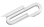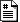Fall 2018

# chapter 3 - numeric types

The good news is that we're not going to do much with that math, and past this we'll mostly be working with other concepts. So if things like sin() and exp() make no sense, just ignore them.

data types

• internal representations
• type() function in python

float numbers are approximate - finite number of bits for storage

>>> 10/3
3.3333333333333335


The last 5 is due to the approximate representation in the computer of the rational number 10/3 , which as a decimal would need an infinite number of digits to store exactly. Python only has a finite number of digits for its 'floating' type.

Integers, on the other hand, can be as big as the computer memory can handle in python. And that's pretty big.

>>> 2**10, 2**20, 2**30, 2**40, 2**300
(1024, 1048576, 1073741824, 1099511627776, 2037035976334486086268445688409378161051468393665936250636140449354381299763336706183397376)


# math library

First let's talk a bit about names, namespaces, and dir()

>>> dir()   # what names are defined ?
['__builtins__', '__doc__', '__name__']
>>> dir(__builtins__)
# ... long list of built-in things ...

>>> from math import *
>>> dir()
# ... another long list of math-y things.


The math functions like sin(), cos(), sqrt(), aren't available in python by default. Instead, you must "import" them from a "module" called "math".

>>> import math
>>> math.sqrt(3.0)


which leaves things from the math module with "math." before their names. If you do things that way, sin(pi/2) is math.sin(math.pi/2).

You can think of math.sqrt as a "sqrt" name "inside" the "math" thing.

>>> import math
>>> dir(math)
['__doc__', '__file__', '__loader__', '__name__', '__package__', '__spec__', 'acos', 'acosh', 'asin', 'asinh', 'atan', 'atan2', 'atanh', 'ceil', 'copysign', 'cos', 'cosh', 'degrees', 'e', 'erf', 'erfc', 'exp', 'expm1', 'fabs', 'factorial', 'floor', 'fmod', 'frexp', 'fsum', 'gamma', 'gcd', 'hypot', 'inf', 'isclose', 'isfinite', 'isinf', 'isnan', 'ldexp', 'lgamma', 'log', 'log10', 'log1p', 'log2', 'modf', 'nan', 'pi', 'pow', 'radians', 'sin', 'sinh', 'sqrt', 'tan', 'tanh', 'tau', 'trunc']


This is actually the beginnings of a topic known as "object oriented programming", which we will dive into soon. Here "math" is the object, and "math.sqrt" is a function within that object.

Be clear about the difference between that and this.

>>> from math import *
>>> dir()
['__annotations__', '__builtins__', '__cached__', '__doc__', '__loader__', '__name__', '__package__', '__spec__', 'acos', 'acosh', 'asin', 'asinh', 'atan', 'atan2', 'atanh', 'ceil', 'copysign', 'cos', 'cosh', 'degrees', 'e', 'erf', 'erfc', 'exp', 'expm1', 'fabs', 'factorial', 'floor', 'fmod', 'frexp', 'fsum', 'gamma', 'gcd', 'hypot', 'inf', 'isclose', 'isfinite', 'isinf', 'isnan', 'ldexp', 'lgamma', 'log', 'log10', 'log1p', 'log2', 'modf', 'nan', 'os', 'pi', 'pow', 'radians', 'sin', 'sinh', 'sqrt', 'sys', 'tan', 'tanh', 'tau', 'trunc']


# accumulating results in a loop

sum = 0
numbers = [1, 10, 20, 18, 17, 34, 22]
for number in numbers:
sum = sum + number
print("The sum is ", sum)


Look at this carefully to understand what's going on. In class: run this. Then put in more print statements to see exactly what is going on during the loop. (The loop is the "for" statement and the indented part after it.)

The text doesn't go into the details of lists until much later - mainly because they have a lot of bells and whistles - but for now let me just say that python lets you collect up things into a sequence, and that these loops happen over such a sequence.

>>> list(range(3))
[0, 1, 2]
>>> type([0, 1, 2])
<type 'list'>

>>> for i in [5, 17, 4]:
...     print(i)
5
17
4


This "accumulator pattern" shows up a lot: we start with a variable which is "empty", and then in a loop "fill it up" with something, accumulating the result.

Here are the types of python values we've seen so far.

• integer : 2, 5, -3
• float : 3.23
• string : "This is a string"
• list : [3, 5, 17]
• built-in function : sin, exp
• module : math

# practice

Write a program to calculate the mean of N numbers input by the user.

• (First ask for N, the ask for the numbers one at a time.)
• Also find the standard deviation.
• Now input the numbers as a python list (i.e. [2, 4, 6]).

The code we wrote in class - average.py - is attached below.

https://cs.marlboro.college /cours /fall2018 /python /notes /chap3
## attachmentslast modified sizeaverage.py Tue Dec 05 2023 09:49 am 438B